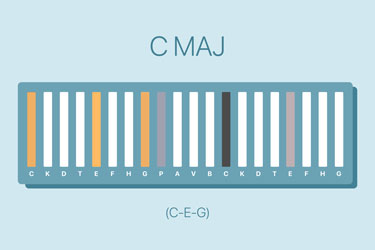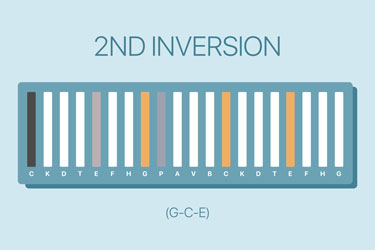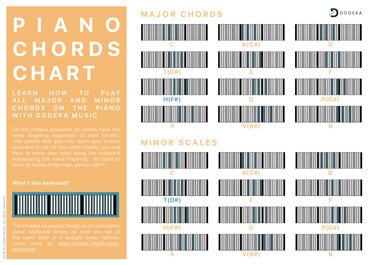# Exploring Piano Chord Inversions On Isomorphic Keyboards

## Play inverted piano chords in a quick and easy way

### Transcript

Hello and welcome to the Dodeka Youtube channel. In today’s piano lesson, we will explore how to invert piano chords and why it is important to know how to use inversions.

In our last lesson about major and minor chords (lesson 3), we learnt about the basic structure of a chord, that is to say, two intervals of 4 and 3 notes (4+3) for major chords and two intervals of 3 and 4 notes for minor chords.

Let's now delve into the concept of chord inversion and discover how to play inverted chords on the piano.

### What is a chord inversion on the piano?

Piano chords inversion is the process of rearranging the notes of harmonic interval into new sequences. In other words, it is a chord whose sound sequence is modified. For example, the C major chord “C-E-G” can be inverted into “E-G-C” and “G-C-E”. Those are the exact same chord. It is only the position of the notes within the chord that are different.The sequence changes - but the notes stays the same.

The number of notes within a chord will determine the number of possible inversions. For example, a 3-note chord, or triad, can be inverted into two different ways. By contrast, a four-note chord has three possible inversions. We refer to them as first, second, etc. inversions.

### First Piano Chord Inversion

To create the 1st chord inversion, all we have to do is move the first note (root note) of the chord to a higher octave. We basically move this note at the end of the chord. Thus, to invert major chords the first formula is: 3-note interval then 5-note interval (3+5), starting from the second note of the basic chord. The first note (or root) is now an octave higher.

Inverting minor chords follows the same principle. Starting from the second note of the basic chord, the inversion formula consists of 4-note interval and 5-note interval (4+5), finishing one octave higher.

### Second Piano Chord Inversion

To create the second inversion, we now want to move the second note of the basic chord at the top of the other notes. So for the 2nd inversion of major chords, the formula is 5-note interval and then 4-note interval or 5+4, starting from the third note of the chord.

Likewise, the formula for the second inversion of minor chords starts on the third note of the minor chord, then one 5-note interval followed by one 3-note interval (5+3).

### Why use inverted chord?

There is no harmonic difference between basic chords and their inversions. So why should we use an inversion rather than a fundamental chord? And how do we know which one to play?

It actually depends.

We can choose to play an inversion instead of the basic chord because we know that the inversion will make us make fewer movements than if we were playing the chord. For example, if we play the series: C major inversion followed by the G major chord. Our hand doesn’t move, only our fingers do. By contrast, if wanted to use the the basic chord in this series, that is, the C major chord. We would have to move our hand along the keyboard by a few keys.

We can also use inversions to emphasise specific notes. For instance, the c major inversion followed by the F major chord will make the C note more prominent. In fact, when we choose an inversion we have to remember that high notes are more prominent.

Finally, we can also choose to play inversions because we prefer the way the inversion sounds.

For example, in his famous track “Song for Guy” , Elton John uses the second inversion of a major chord, the first inversion of a minor chord, and then the first inversion of the same major chord.

### Practice your chords with easyChords

Explore and learn all piano chords with easyChords - an interactive web app where you can see and hear the chords.

### Key takeaways

• Inversion is the process of rearranging the notes composing basic chords, that is, to put the notes an octave higher.

• For 3-note chords there are two inversions possible.

• From a harmonic viewpoint, basic chords and inversions are the same.

• We invert piano chords for three reasons. (1) to make less movements with our hand, (2) to emphasise particular notes, or (3) to play sounds that we prefer.

### Useful Resources

#### PDF Piano Chords Chart

###### PDF & Printable Piano Chord Chart

Tired of learning piano chords? Try our piano chord chart and learn all major and minor piano chords in a quick and simple way. Get our free chart.#### How To Learn & Play Piano Chords Quicker

###### Blog article

Struggling with chords? Learn how piano chords can become easy to remember, quick to learn and simple to play with Dodeka Music.